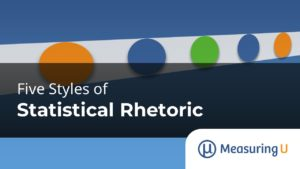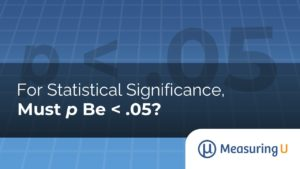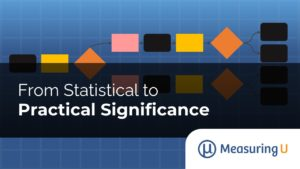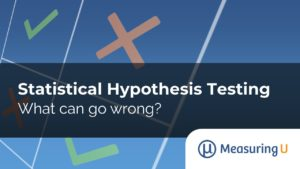### Five Styles of Statistical Rhetoric

When learning statistics, you’ll encounter many formulas based on principles of probability and mathematics. But statistics isn’t just a formulaic process where you enter data and are told what to do. Statistics should guide, not dictate, decisions. In making decisions, though, there are different styles of interpreting data. Although a lot of people think statistics### For Statistical Significance, Must p Be < .05?

If you know even just a little about statistics, you know that the value .05 is special. When the p-value obtained from conducting a statistical test falls below .05, it typically gets a special designation we call statistically significant. This is the conventional threshold for publishing findings in academic journals, and consequently, it is ascribed### From Statistical to Practical Significance

Hypothesis testing is one of the most common frameworks for making decisions with data in both scientific and industrial contexts. But this statistical framework, formally called Null Hypothesis Statistics Testing (NHST), can be confusing (and controversial). In an earlier article, we showed how to use the core framework of statistical hypothesis testing: you start with